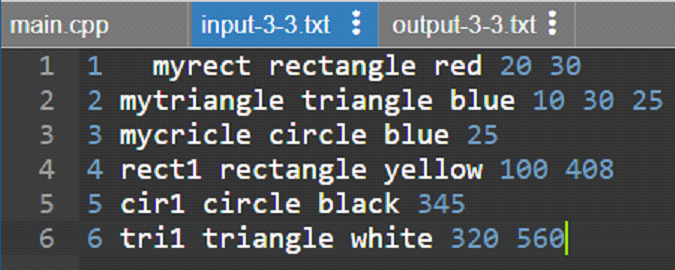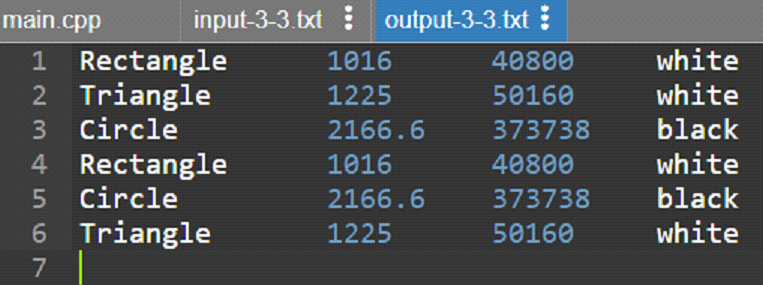# Can someone help me with this? It’s C++. I’m uploading the requirements the professor is asking for.

## Question

Can someone help me with this? It’s C++. I’m uploading the requirements the professor is asking for. The only “required” library I have to include is iostream, but it’s possible to use any other library in addition if needed. Just in case if needed, we’re using Visual Studio, but I don’t think that’s a piece of relevant information for the assignment.

1. Write a class of Shape that has two member variables, perimeter and area, and pure virtual functions to calculate the perimeter and area of the shape. It also contains two additional member variables:

a. m_name: a string with no more than 10 characters for the name of the object

b. m_ID: an integer index of the object.

2. Write a class of Color with a member variable that can be red, yellow, blue, black, and white.

3. Write three classes for rectangle, triangle, and circle objects, derived from the class of Shape and Color for objects with the shape of the triangle, rectangle, or circle, and any of the colors defined in the class of Color.

4. Write necessary constructor/destructor functions, accessor/mutator functions for each class.

a. print: print the pertinent information for a given shape and color

b. calculate: calculate the area and perimeter for the current shape

5. Your program should read the object info from an input file called input-3-3.txt, which lists the index, object name, color, length of each side (radius if it is a circle). similar as follows:

1  myrect  rectangle  red  20  30

2  mytriangle  triangle  blue  10  30  35

3  mycircle  circle  blue  25

4  rect2  rectangle   blue  30  40

6. Your program needs to calculate the perimeter and area of each object, and set the object’s color to be black if its area is larger than the overall average of all objects and white otherwise. Your program should output the following information to another file called output-3-3.txt. Please name your main file as prog-3-3.cpp.

a. The overall average area of all objects

b. The index, name, perimeter, area, color

The other picture below is the output that the program should give. I believe the answer just has to look similar to the one below, so it doesn’t need to be exactly the same. It needs to follow the same structure.

1     myrect          rectangle      red        20         30
2     mytriangle    triangle         blue       10         30      35
3     mycircle       circle             blue       25
4     rect1            rectangle       yellow    100      408
5      rect2           rectangle       blue        302     40
6     cir1              circle              black      345
7     tri1               triangle          white      320      560    280
8     tri2               triangle          red         45        87      63
9     cir2              circle              red         78
10   cir4              circle              yellow    43

## Summary

Basically, we have defined a base class shape with a virtual function show_area, and another function set_dim, which are redefined by all the other derived classes of shape. They are rectangle, circle, and triangle. Each class has its own definition for the area. The major thing done, is to read the input for each shape from an input file named ‘input-3-3.txt’ and write the details of the area to an output file.

## Explanation

We have defined the class Shape two protected data members with the names x and y. And, we have defined function set_dim to set the dimensions of the shape. We have defined the function set_area to find the area of each shape and we have made it virtual. The function set_perimeter is defined to find the perimeter of each shape and it is made virtual. And, the function display is defined to display details of each shape and it is made virtual. The derived class rectangle, circle, and triangle are defined which have a copy of the protected variables of the Shape class. All these classes have their specific definition for the show_area with the required formula to calculate the area. A file named ‘input-3-3.txt’ is opened and each record is read to detect the shape, and according to that class, the object calls the set_dim function and specifies the dimensions. The output file is ‘output-3-3.txt’. The color of each shape is set to black if the average area is less than that shape area otherwise white.

## Code

#include <iostream>
#include<fstream>
#include<string>
using namespace std;
#include <cmath>
#include <iomanip>
/* base class with virtual function */
class Shape {
protected: double x, y,z;
public:
double perimeter,area; string m_name; string color;
void set_dim(double i, double j=0,double k=0) { x = i; y = j; z=k;}
virtual void show_area() {
cout << "No area computation defined ";
cout << "for this class.\n"; }
/* to calculate perimeter */
virtual void show_perimeter(){
}
/* to display */
virtual void display(ofstream& out){
}
};
/* to implement Color class */
class Color{
public: string color1;
Color(){}
};
/* first derived class for shape triangle */
class triangle : public Shape, public Color {
public:
/* to calculate area */
void show_area() {
double s=(x+y+z)/2.0;
area=sqrt(s*(s-x)*(s-y)*(s-z));
}
/* to calculate perimeter */
void show_perimeter(){
perimeter=x+y+z;
}
/* to display */
void display(ofstream &out){
out<<left; out<<setw(15)<<m_name<<setw(10)<<perimeter<<setw(10)<<area;
out<<setw(10)<<color<<endl;
}
};
/* second derived class for shape rectangle */
class rectangle : public Shape, public Color{
public:
/* to calculate area */
void show_area() {
area=x*y;
}
/* to calculate perimeter */
void show_perimeter(){
perimeter=2*(x+y);
}
/* to display */
void display(ofstream &out){
out<<left; out<<setw(15)<<m_name<<setw(10)<<perimeter<<setw(10)<<area;
out<<setw(10)<<color<<endl;
}
};
/* thrid derived class for shape circle */
class circle : public Shape, public Color{
public:
/* to calculate area */
void show_area() {
area=3.14*x*x;
}
/* to calculate perimeter */
void show_perimeter(){
perimeter=2*3.14*x;
}
/* to display */
void display(ofstream &out){
out<<left; out<<setw(15)<<m_name<<setw(10)<<perimeter<<setw(10)<<area;
out<<setw(10)<<color<<endl;
}
};

int main(void) {
Shape *p;
triangle t;
rectangle r;
circle c;
/* to read the input file */
ifstream in; in.open("input-3-3.txt");
/* to write the output file */
ofstream out; out.open("output-3-3.txt");
int x; int cc=0; double avg=0.0;
while(in>>x){
string object;  in>>object;
string shape; in>>shape;
string color; in>>color;
/* for rectangle */
if(shape=="rectangle"){
int x,y; in>>x>>y;
p[cc]=&r; p[cc]->set_dim(x,y); p[cc]->show_area(); p[cc]->show_perimeter();
avg+=(p[cc]->area); p[cc]->m_name="Rectangle";
}
/* for circle */
else if(shape=="circle"){
int r; in>>r;
p[cc]=&c; p[cc]->set_dim(r); p[cc]->show_area(); p[cc]->show_perimeter();
avg+=(p[cc]->area); p[cc]->m_name="Circle";
}
/* for triangle */
else if(shape=="triangle"){
int a,b,c; in>>a>>b>>c;
p[cc]=&t; p[cc]->set_dim(a,b,c); p[cc]->show_area(); p[cc]->show_perimeter();
avg+=(p[cc]->area); p[cc]->m_name="Triangle";
}
cc++;
}
/* to calculate average area */
for(int i=0;i<cc;i++){
avg+=p[i]->area; } avg=avg/6.0;
for(int i=0;i<cc;i++){
if(p[i]->area>avg) p[i]->color="black";
else p[i]->color="white"; p[i]->display(out);
} }


## Output